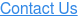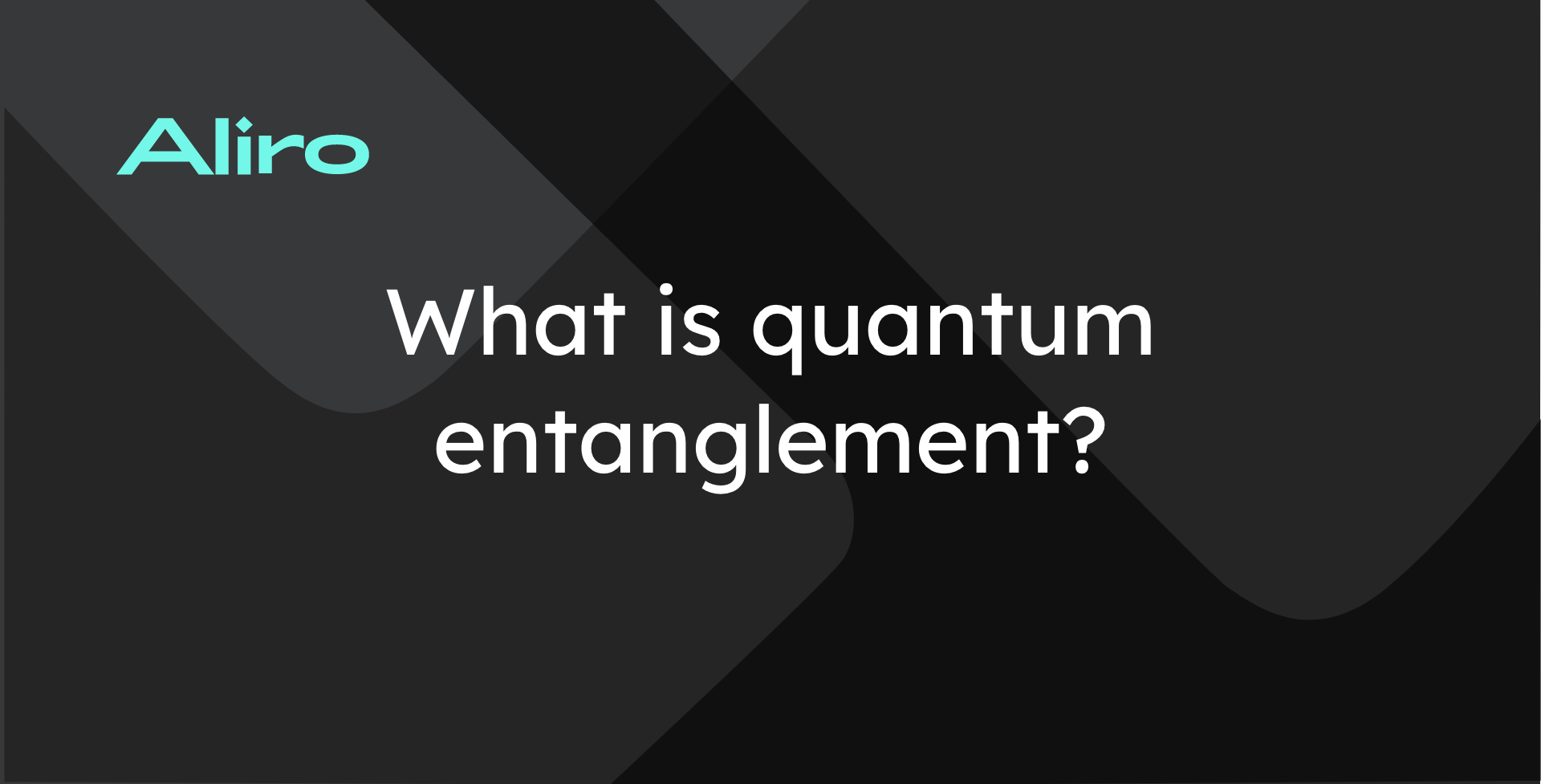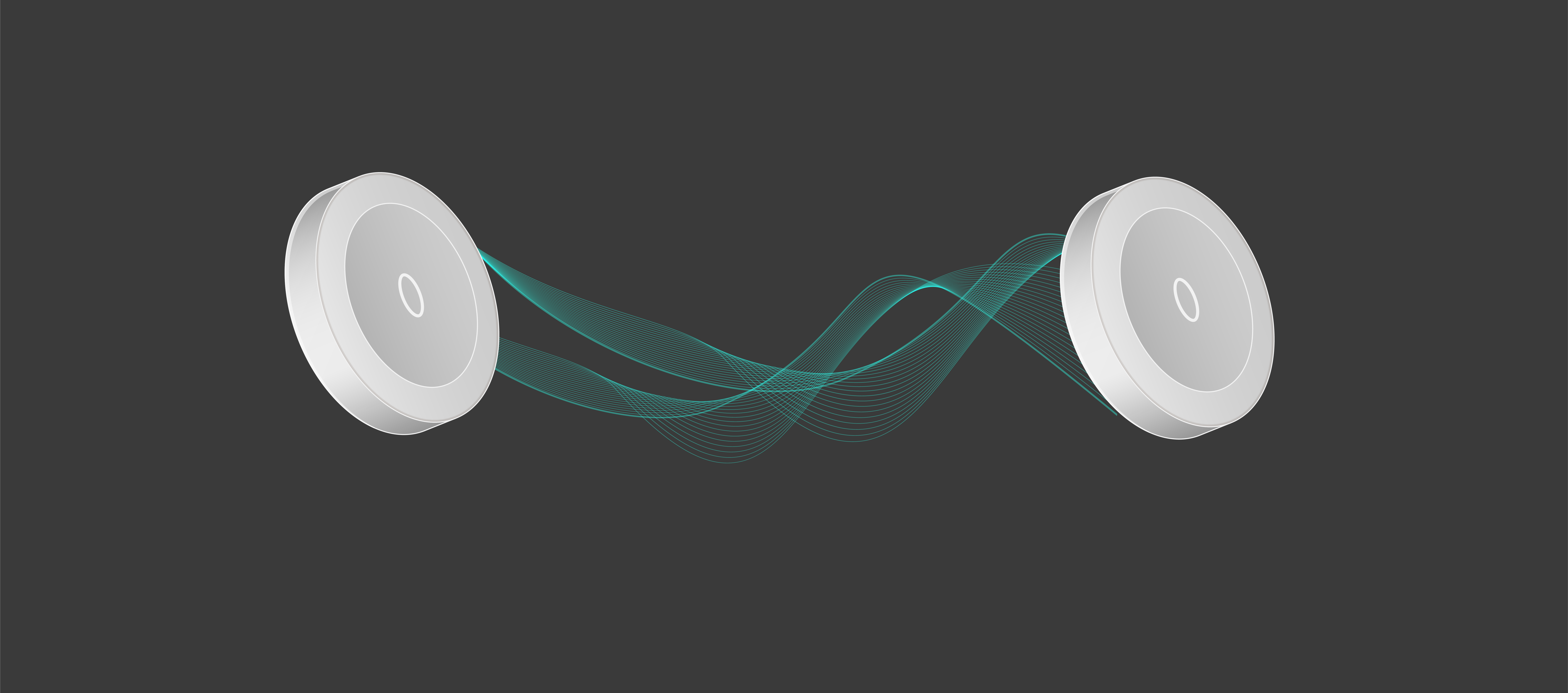# What is quantum entanglement?“Entanglement is iron to the classical world’s bronze age.” - Michael Nielsen and Isaac Chuang in Quantum Computation and Quantum Information

The primary job of a quantum network is to distribute entanglement. This may sound surprising, since we are used to thinking of networks as tools for transmitting data, first and foremost. To understand the power of quantum networks, we first need to understand the basics of how entanglement actually works. In this post, which assumes no background in quantum communication, we will take a look at what entanglement is and how it enables the exciting applications of quantum networks. By understanding entanglement a bit better, you will be better prepared to harness the power of the coming generation of quantum networks.

## Quantum states and superposition

For starters, let’s take a look at one of the fundamental building blocks of quantum communication: the qubit. Quantum data is stored in probabilistic qubits, as opposed to bits, which store non-quantum or “classical” data. Unlike a classical bit, which must be in one of two states, 0 or 1, a qubit can be in a probabilistic distribution of both states, known as a superposition. However, when we try to check what state a qubit is in by measuring, we always find it in one of two states, which we can again call 0 or 1. This is one of the tricky aspects of quantum mechanics -- we can’t view the actual quantum state of a qubit. Instead, when we measure a qubit, nature seems to randomly sample one of the two states from the qubits probability distribution. This random selection of either a 0 or a 1 is known as “collapsing.”Measuring qubits in an equal superposition is like flipping a fair coin

To see how this works, imagine we have two qubits that are each in an “equal superposition,” meaning their probability distribution is evenly distributed between 0 and 1. In other words, there is a 50-50 chance of measuring each. In this way, the qubits are similar to two coins that are being flipped. While they are in the air, we don’t know how they will land. But once we catch them in our hand, we will find them lying as either heads or tails.

## Getting entangled

Now, things get a bit weirder when two qubits have a chance to interact with each other. When this happens, the probability distributions of the two qubits can become intertwined. The qubits can become dependent on each other. In this case, knowing the measurement of one qubit could tell you something about what will happen when you measure the other qubit. This kind of connection between the probability distributions, or correlation between the measurement outcomes, is exactly what we mean by entanglement.

Take, for example, two qubits that are entangled in a special state, known as a Bell state, named after the physicist that discovered many of the surprising properties of entanglement, John Bell. In this kind of state, each qubit on it's own still has equal chances of resulting in either a 0 or a 1 when measured, as before. But the qubits now have the strange property of always resulting in the same outcome. So, if we measure one qubit first, there is a 50% chance of either a 0 or a 1. But, once we see the outcome, we know with 100% certainty that the other qubit will have the same result. This should seem a bit strange! Comparing this to our normal understanding of the world reveals just how strange it is.When two qubits are entangled, their outcomes are dependent on each other

Coming back to the analogy of the two coins, let’s see if we can find a similar analogy for two qubits that are entangled in a Bell state. Imagine again our two coins spinning in the air simultaneously. But this time, when we catch one out of the air first, its result determines the result of the other coin that is still spinning! Clearly this doesn’t seem right. In fact, physicists, beginning with John Bell, have shown that there is no way to achieve this kind of correlation without using quantum mechanical systems -- systems that have entanglement.

If this all seems a bit confusing or magical, you are in good company! Einstein famously rejected the idea of entanglement. However, while it may be hard to wrap our heads around, experimental evidence has shown again and again that particles do actually become entangled in nature. Quantum computers and networking devices allow us to use that entanglement to our advantage.

In a future post, we will dig deeper into the details of how entanglement is actually generated and methods for distributing entanglement across the globe using quantum networks.

## Quantum Entanglement as a Service

So, why do we want to distribute entanglement across the globe? In short, we can take advantage of the fact that entanglement allows for correlations beyond what is possible classically to enable new applications that are not possible classically. This allows for global Entanglement as a Service (EaaS).

For example, entanglement enables a new kind of security that is backed by physics. Entanglement also allows for transporting qubits, solving larger problems by connecting quantum computers, higher accuracy GPS systems, and more.

The unique capabilities of entanglement -- the correlations it enables -- make it a unique resource. Using this resource, users on a network can achieve any number of applications. Quantum networks built with quantum repeaters allow this resource to be shared across the globe.